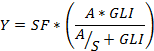SORTIE-ND
Software for spatially-explicit simulation of forest dynamics

# Absolute growth behaviors

Several behaviors apply an absolute growth version of the Michaelis-Menton function.

### Parameters for these behaviors

Parameter nameDescription
Adult Constant Area Growth in sq. cm/yrThe constant amount of basal area by which to increase a tree's basal area. Applies to basal-area-increment-limited behaviors.
Asymptotic Diameter Growth (A)Asymptote of the Michaelis-Menton growth function at high light - function term A below.
Slope of Growth Response (S)Slope of the Michaelis-Menton growth function at zero light - function term S below.
Length of Current Release FactorControls the magnitude of the effects of release.
Length of Last Suppression FactorControls the magnitude of the effects of suppression.
Mortality Threshold for SuppressionDefines the growth rate for suppressed status in terms of tree mortality. The value is expressed as the proportion of trees which die at the growth rate which defines suppressed status, expressed as a fraction between 0 and 1. For instance, if this value is 0.1, the growth rate for suppressed status is one at which 10% of trees die with that growth.
Years Exceeding Threshold Before a Tree is SuppressedThe number of years for which a tree's growth must be below the defined suppression threshold before it is considered to be suppressed.

Absolute growth is calculated with the equation:where

• Y = log10(radial growth + 1)
• SF is the suppression factor
• A is the Asymptotic Diameter Growth (A) parameter
• S is the Slope of Growth Response (S) parameter
• GLI is the global light index, calculated by a light behavior

Amount of diameter growth per timestep is calculated as

growth = (((10Y - 1) * 2 )/ 10) * T

where T is the number of years per timestep.

The absolute growth behaviors also take into account suppression status. A tree is considered suppressed if its growth rate for the previous timestep falls below a certain threshold. That threshold is the rate of growth at which X% of juveniles die, where X is a user-settable parameter. The threshold is calculated for each species by solving the BC mortality equation for G (growth), where m is the threshold growth rate.

A tree's suppression state is a multiplicative factor in its growth rate. If a tree is not suppressed, the suppression factor in the growth equation is set to 1 (no effect on growth). If the tree is suppressed, the suppression factor is calculated as follows:

SF = e((g*YLR) - (d*YLS))

where:

• SF is the suppression factor
• g is the Length of Current Release Factor parameter
• YLR is the length of the last (or current) period of release, in years
• d is the Length of Last Suppression Factor parameter
• YLS is the length of the last (or current) period of suppression, in years

Details of this model are published in Wright et al 2000.

## Absolute growth limited to radial increment

### How it works

This behavior calculates an amount of diameter growth according to the absolute growth equation. Growth is limited to a maximum of the constant radial increment for the species of tree to which it is being applied. The increment is calculated as described in the "Constant radial growth" behavior. Note that the increment parameter specifies radial growth; the behavior makes all necessary conversions.

### How to apply it

This behavior can be applied to seedlings, saplings, and adults of any species. Any tree species/type combination to which it is applied must also have a light behavior applied. You can use either the diam with auto height or diam only version.

## Absolute growth limited to basal area increment

### How it works

This behavior calculates an amount of diameter growth according to the absolute growth equation. Growth is limited to a maximum of a constant basal area increment. The amount of diameter increase is calculated by dividing the annual basal area increment of the tree's species by the diameter of the tree. The increment is calculated as described in the "Constant basal area growth" behavior.

### How to apply it

This behavior can be applied to seedlings, saplings, and adults of any species. Any tree species/type combination to which it is applied must also have a light behavior applied. You can use either the diam with auto height or diam only version.

## Non-limited absolute growth - diam with auto height

### How it works

The amount of diameter increase returned by the absolute growth equation is applied to the tree.

### How to apply it

This behavior can be applied to seedlings, saplings, and adults of any species. Any tree species/type combination to which it is applied must also have a light behavior applied. You can use either the diam with auto height or diam only version.